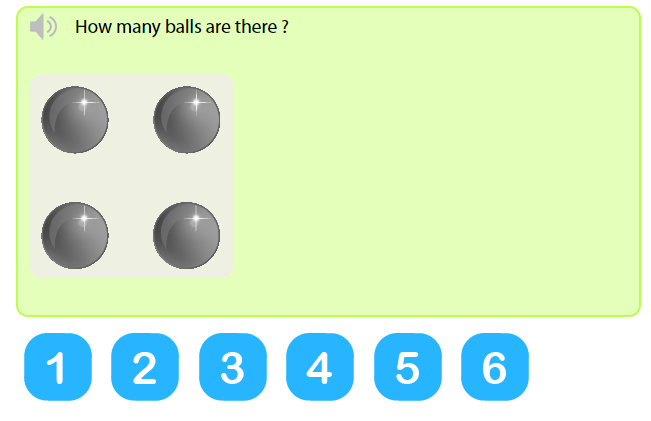# Learning numbers 1 to 6 - Count to 6 test online

## 1 to 6 counting activity for kids

Learning numbers 1 to 6 is a perfect way to reinforce the understanding of different numerals 1, 2, 3, 4, 5, 6 to kids.• ## IN THE SAME CATEGORY

### Important fact about our learning numbers 1 to 6 practice for kids

As we continue to gradually learn how to count, we come across a new number every day. That said, count to 6 test online is an excellent counting skill interactive enough, thus providing much joy to kids.

Moreover, as challenging as it is, kids become so much engaged in the counting concept as they repeatedly count using several different methods.

In view of this, we have a wide range of fun 1 to 6 counting activity for kids that will greatly improve their counting skills 1 to 6.

We have made it possible in our 1 to 6 counting activity for kids to be able to develop a strong mathematical foundation and understanding of numbers. Playing with different sets of objects and asking kids to determine how many objects are there per set will help them develop an appropriate sense of quantity.

### Example - How many balls are there?

Given our exercises, our sets of objects will differ in terms of numbers. So our task here will be to tell how many balls are there in each set.The way thes balls below are dispose look like a dice and we will enable you easily determine how many balls are there.

This is because the numeral quantity of each balls is written just down the set of balls. So as you finish counting the number of balls, you’ll also count the numbers down, so as to find the corresponding number of balls.

• Now, we begin by counting the balls. Let’s go…. 1, 2, 3, 4
• Secondly, we select the number 4 on the number line.

As there are 4 balls, I have also counted to 4. As I look up above that particular number, I can see the number 4, boldly written ‘4’.

Therefore, there are 4 balls

•